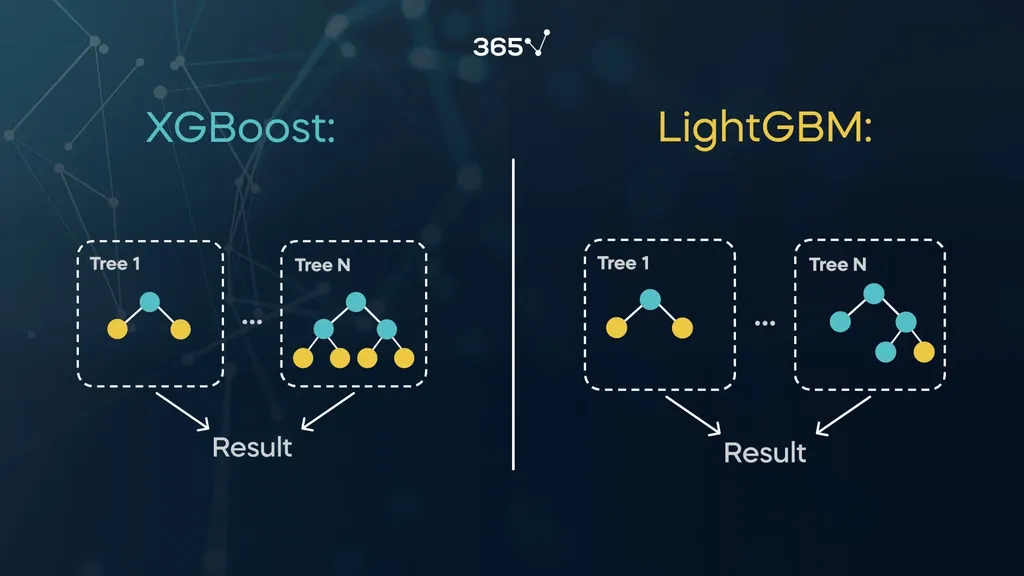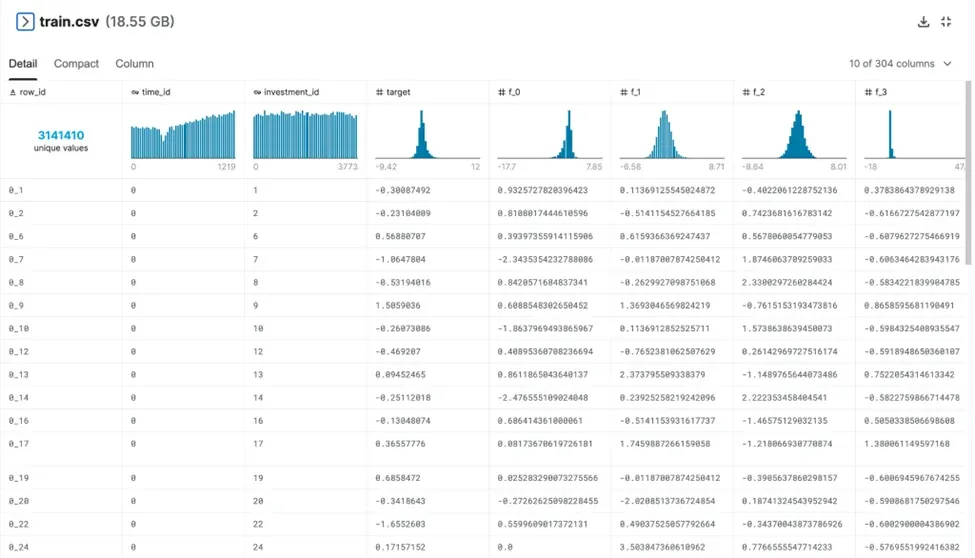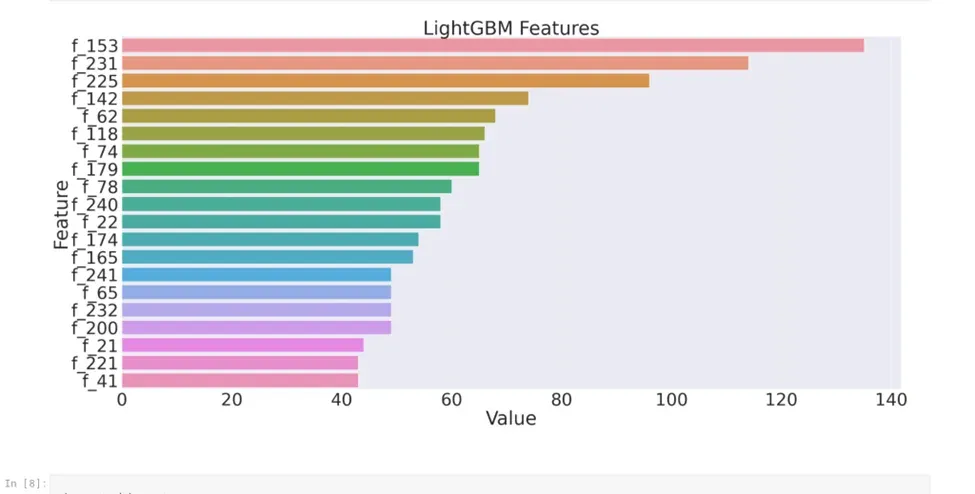# How to Use XGBoost and LGBM for Time Series Forecasting?

In time series forecasting, a machine learning model makes future predictions based on old data that our model trained on. It is arranged chronologically, meaning that there is a corresponding time for each data point (in order). Of course, there are certain techniques for working with time series data, such as XGBoost and LGBM.

In this tutorial, we will go over the definition of gradient boosting, look at the two algorithms, and see how they perform in Python.

Gradient boosting is a machine learning technique used in regression and classification tasks. It creates a prediction model as an ensemble of other, weak prediction models, which are typically decision trees. Essentially, how boosting works is by adding new models to correct the errors that previous ones made.

It is worth noting that both XGBoost and LGBM are considered gradient boosting algorithms. While there are quite a few differences, the two work in a similar manner.

## What Is XGBoost?

XGBoost is a type of gradient boosting model that uses tree-building techniques to predict its final value. It usually requires extra tuning to reach peak performance.

As the XGBoost documentation states, this algorithm is designed to be highly efficient, flexible, and portable. Moreover, it is used for a lot of Kaggle competitions, so it’s a good idea to familiarize yourself with it if you want to put your skills to the test.

## What is LGBM?

The light gradient boosting machine algorithm – also known as LGBM or LightGBM – is an open-source technique created by Microsoft for machine learning tasks like classification and regression. It is quite similar to XGBoost as it too uses decision trees to classify data.

One of the main differences between these two algorithms, however, is that the LGBM tree grows leaf-wise, while the XGBoost algorithm tree grows depth-wise:In addition, LGBM is lightweight and requires fewer resources than its gradient booster counterpart, thus making it slightly faster and more efficient.

## Gradient Boosting with LGBM and XGBoost: Practical Example

In this tutorial, we’ll show you how LGBM and XGBoost work using a practical example in Python.

The dataset we’ll use to run the models is called Ubiquant Market Prediction dataset. It was recently part of a coding competition on Kaggle – while it is now over, don’t be discouraged to download the data and experiment on your own!

Please note that this dataset is quite large, thus you need to be patient when running the actual script as it may take some time.

The Ubiquant Market Prediction file contains features of real historical data from several investments:Keep in mind that the f_4 and f_5 columns are part of the table even though they are not visible in the image.

In this example, we have a couple of features that will determine our final target’s value. The main purpose is to predict the (output) target value of each row as accurately as possible. It is worth mentioning that this target value stands for an obfuscated metric relevant for making future trading decisions.

We will do these predictions by running our .csv file separately with both XGBoot and LGBM algorithms in Python, then draw comparisons in their performance.

## How to Run an XGBoost Model in Python?

Let’s see how an XGBoost model works in Python by using the Ubiquant Market Prediction as an example.

### Step 1: Import The Necessary Python Libraries

First, you need to import all the libraries you’re going to need for your model:

import pandas as pd
import numpy as np
import matplotlib.pyplot as plt
from pathlib import Path
import os
from tqdm import tqdm
import random
import seaborn as sns
import math
import warnings
warnings.filterwarnings('ignore')
from sklearn.model_selection import train_test_split
import xgboost as xgb
import gc


As you can see, we’re importing the pandas package, which is great for data analysis and manipulation. Additionally, there’s also NumPy, which we’ll use to perform a variety of mathematical operations on arrays.

Don’t forget about the train_test_split method – it is extremely important as it allows us to split our data into training and testing subsets.

Last, we have the xgb.XGBRegressor method which is responsible for ensuring the XGBoost algorithm’s functionality. It is imported as a whole at the start of our model.

### Step 2: Define the Path for the Dataset

By using the Path function, we can identify where the dataset is stored on our PC. In case you’re using Kaggle, you can import and copy the path directly.

Note that the following contains both the training and testing sets:

DATA_PATH = Path('../input/ump-train-picklefile')
SAMPLE_TEST_PATH = Path('../input/ubiquant-market-prediction')


### Step 3: Reduce Memory Usage

In most cases, there may not be enough memory available to run your model. For this reason, you have to perform a memory reduction method first. In this case, I’ve used a code for reducing memory usage from Kaggle:

def reduce_mem_usage(df):
""" iterate through all the columns of a dataframe and modify the data type
to reduce memory usage.
"""
start_mem = df.memory_usage().sum() / 1024**2
print('Memory usage of dataframe is {:.2f} MB'.format(start_mem))

for col in df.columns:
col_type = df[col].dtype

if col_type != object:
c_min = df[col].min()
c_max = df[col].max()
if str(col_type)[:3] == 'int':
if c_min > np.iinfo(np.int8).min and c_max <                  np.iinfo(np.int8).max:
df[col] = df[col].astype(np.int8)
elif c_min > np.iinfo(np.int16).min and c_max < np.iinfo(np.int16).max:
df[col] = df[col].astype(np.int16)
elif c_min > np.iinfo(np.int32).min and c_max < np.iinfo(np.int32).max:
df[col] = df[col].astype(np.int32)
elif c_min > np.iinfo(np.int64).min and c_max < np.iinfo(np.int64).max:
df[col] = df[col].astype(np.int64)
else:
if c_min > np.finfo(np.float16).min and c_max < np.finfo(np.float16).max:
df[col] = df[col].astype(np.float16)
elif c_min > np.finfo(np.float32).min and c_max < np.finfo(np.float32).max:
df[col] = df[col].astype(np.float32)
else:
df[col] = df[col].astype(np.float64)
else:
df[col] = df[col].astype('category')

end_mem = df.memory_usage().sum() / 1024**2
print('Memory usage after optimization is: {:.2f} MB'.format(end_mem))
print('Decreased by {:.1f}%'.format(100 * (start_mem - end_mem) / start_mem))

return df

While the method may seem complex at first glance, it simply goes through your dataset and modifies the data types used in order to reduce the memory usage.

Next, we will read the given dataset file by using the pd.read_pickle function. We will insert the file path as an input for the method. After, we will use the reduce_mem_usage method we’ve already defined in order. This can be done by passing it the data value from the read function:

train = pd.read_pickle(DATA_PATH/'train.pkl')
train = reduce_mem_usage(train)
gc.collect()


### Step 4: Clean and Split the Dataset

To clear and split the dataset we’re working with, apply the following code:

train.drop(['row_id', 'time_id'], axis=1, inplace=True)
X = train.drop(['target'], axis=1)
y = train["target"]
X_train, X_valid, y_train, y_valid = train_test_split(X, y, test_size=0.01, random_state=42, shuffle=False)


Our first line of code drops the entire row and time columns, thus our XGBoost model will only contain the investment, target, and other features.

In the second and third lines, we divide the remaining columns into an X and y variables. The former will contain all columns without the target column, which goes into the latter variable instead, as it is the value we are trying to predict.

Then it’s time to split the data by passing the X and y variables to the train_test_split function.

### Step 5: Delete Unneeded Data to Further Reduce Memory Usage

Now, you may want to delete the train, X, and y variables to save memory space as they are of no use after completing the previous step:

del train
del X
del y


Note that this will be very beneficial to the model – especially in our case since we are dealing with quite a large dataset.

### Step 6: Employ the XGBoost Algorithm

We will use the XGBRegressor() constructor to instantiate an object. It can take multiple parameters as inputs – each will result in a slight modification on how our XGBoost algorithm runs.

We will list some of the most important XGBoost parameters in the tuning part, but for the time being, we will create our model without adding any:

model = xgb.XGBRegressor()

### Step 7: Run the XGBoost Model

The fit function requires the X and y training data in order to run our model. Moreover, we may need other parameters to increase the performance. For this reason, I’ve added early_stopping_rounds=10, which stops the algorithm if the last 10 consecutive trees return the same result.

model.fit(X_train, y_train, early_stopping_rounds=10, eval_set=[(X_valid, y_valid)], verbose=1)

### Step 8: Tune the XGBoost Model

Model tuning is a trial-and-error process, during which we will change some of the machine learning hyperparameters to improve our XGBoost model’s performance.

We can do that by modifying the inputs of the XGBRegressor function, including:

1. max_depth: by giving a defined max depth of 12, the algorithm will not create more than 12 levels in each tree.
2.  n_estimators: the number of trees in the model.
3. learning_rate: the learning speed of our algorithm. In our case, it is to equal 0.03. You can play around with this value until you reach the perfect rate.
4. subsample: the fraction of observations to be randomly sampled for each tree.
5. tree_method: allows you to choose the tree construction algorithm. Some other choices include hist, and approx.
model = xgb.XGBRegressor(
n_estimators=1000,
learning_rate=0.03,
max_depth=12,
subsample=0.9,
colsample_bytree=0.7,
missing=-999,
random_state=1111,
tree_method='gpu_hist'
)


Feel free to browse the documentation if you’re interested in other XGBRegressor parameters.

## How to Run an LGBM Model in Python?

Let’s see how the LGBM algorithm works in Python, compared to XGBoost. You’ll note that the code for running both models is similar, but as mentioned before, they have a few differences.

### Step 1: Import the Necessary Python Libraries

We will need to import the same libraries as the XGBoost example, just with the LGBMRegressor function instead:

from lightgbm import LGBMRegressor

Steps 2,3,4,5, and 6 are the same, so we won’t outline them here. Please ensure to follow them, however, otherwise your LGBM experimentation won’t work.

### Step 7: Run the LGBM Model

Once all the steps are complete, we will run the LGBMRegressor constructor. You can also view the parameters of the LGBM object by using the model.get_params() method:

model = LGBMRegressor()
model.get_params()


As with the XGBoost model example, we will leave our object empty for now.

Note that there are some differences in running the fit function with LGBM. In this case, we have double the early_stopping_rounds value and an extra parameter known as the eval_metric:

model.fit(X_train, y_train,
eval_set=[(X_valid, y_valid)],
verbose=50,
eval_metric='rmse',
early_stopping_rounds=20)


### Step 8: Tune the LGBM Model

As previously mentioned, tuning requires several tries before the model is optimized. Once again, we can do that by modifying the parameters of the LGBMRegressor function, including:

1. objective: the learning objective of your model.
2. boosting_type: the traditional gradient boosting decision tree as our boosting type.
3. num_leaves: the maximum number of tree leaves.

Check out the algorithm’s documentation for other LGBMRegressor parameters.

model = LGBMRegressor(
objective="regression",
metric="l2",
boosting_type="gbdt",
n_estimators=1400,
num_leaves=100,
max_depth=10,
learning_rate=0.05,
subsample=0.8)


### Step 9: Plot the LGBM Features Depending on Significance

We have trained the LGBM model, so what’s next? Well, now we can plot the importance of each data feature in Python with the following code:

def plotImp(model, X , num = 20, fig_size = (40, 20)):
feature_imp = pd.DataFrame({'Value':model.feature_importances_,'Feature':X.columns})
plt.figure(figsize=fig_size)
sns.set(font_scale = 5)
sns.barplot(x="Value", y="Feature", data=feature_imp.sort_values(by="Value",
ascending=False)[0:num])
plt.title('LightGBM Features')
plt.tight_layout()
plt.savefig('lgbm_importances-01.png')
plt.show()

plotImp(model, X_valid)


As a result, we obtain this horizontal bar chart that shows the value of our features:## How to Measure XGBoost and LGBM Model Performance in Python?

To measure which model had better performance, we need to check the public and validation scores of both models.

### Public Scores

Public scores are given by code competitions on Kaggle. They rate the accuracy of your model’s performance during the competition's own private tests.

While these are not a standard metric, they are a useful way to compare your performance with other competitors on Kaggle’s website.

In our case, the scores for our algorithms are as follows:

• LGBM model:1330
• XGBoost model:1380

### Validation Scores

Here is how both algorithms scored based on their validation:

• LGBM model: 92033
• XGBoost model: 92008

### Comparing the Results

Let’s compare how both algorithms performed on our dataset. While the XGBoost model has a slightly higher public score and a slightly lower validation score than the LGBM model, the difference between them can be considered negligible.

In practice, you would favor the public score over validation, but it is worth noting that LGBM models are way faster – especially when it comes to large datasets.

In conclusion, factors like dataset size and available resources will tremendously affect which algorithm you use.

## XGBoost and LGBM for Time Series Forecasting: Next Steps

XGBoost and LGBM are trending techniques nowadays, so it comes as no surprise that both algorithms are favored in competitions and the machine learning community in general. Due to their popularity, I would recommend studying the actual code and functionality to further understand their uses in time series forecasting and the ML world.

If you are interested to know more about different algorithms for time series forecasting, I would suggest checking out the course Time Series Analysis with Python. The 365 Data Science program also features courses on Machine Learning with Decision Trees and Random Forests, where you can learn all about tree modelling and pruning.

The entire program features courses ranging from fundamentals for advanced subject matter, all led by industry-recognized professionals. If you want to see how the training works, start with a selection of free lessons by signing up below.

Learn data science with industry experts